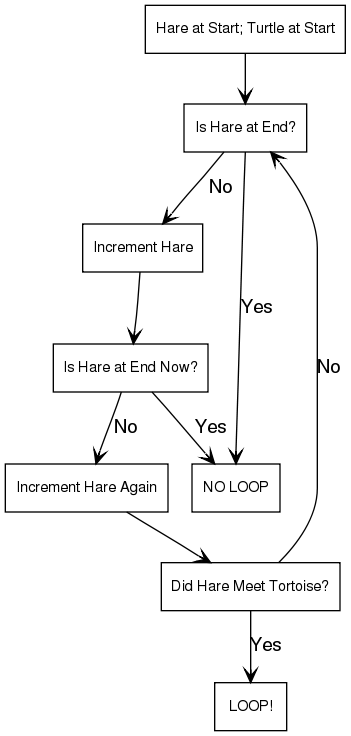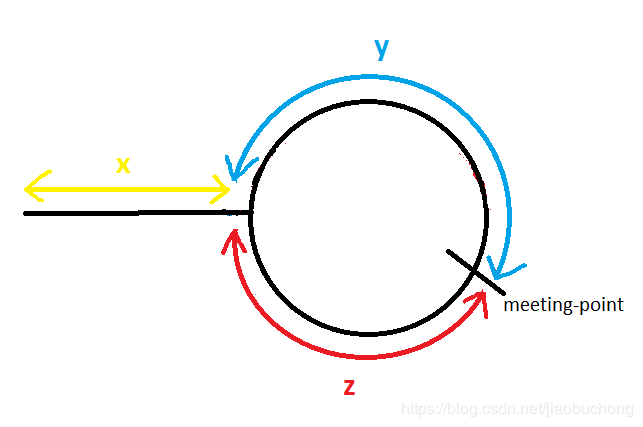# [LeetCode From Day One] - Floyd Cycle Detection

Floyd环路检测算法，又称龟兔赛跑算法（Tortoise and Hare Algorithm），是Robert W. Floyd在上世纪60年代发明的在线性时间内检测单链表，有限状态机（FSM）或者迭代函数中是否存在环路的算法。最简单的一个检测环路的方法就是遍历链表，记录已被访问的节点，如果某个节点的访问次数超过1，则表明存在环路，时间复杂度为\$O(n^2)\$。而Floyd算法可以在线性时间内检测环路。其算法基本思想是如果链表存在环路，那么在环上以一快一慢不同速度前进的两个指针一定会相遇，并且算法可以求出相遇处所在环的起点与长度。下图是一个简单的算法示意图。（图片Credit：Floyd’s Cycle Detection Algorithm (The Tortoise and the Hare)）龟兔赛跑可能是最著名的环路检测算法，同时也是一个非常直观的例子。`Tortoise``Hare`是两个指针，同时从链表的头部出发。每次迭代，`Tortoise`爬得比较慢，只能往前走一步，`Hare`则能前进两步。如果链表存在环，`Hare`最终会绕圈跑，可能不止一圈，但是在`Tortoise`进入这个loop之后，`Hare`最终都会与`Tortoise`相遇。如果单链表中无环，显然`Hare`会率先达到链表末尾，算法退出。算法时间复杂度为\$O(n)\$，空间复杂度为常数，\$O(1)\$。# Happy Number

Input: 19
Output: true
Explanation:
\$1^2+9^2=82\$
\$8^2+2^2=68\$
\$6^2+8^2=100\$
\$1^2+0^2+0^2=1\$

``````class Solution {
public int sumOfSquares(int n) {
int sum = 0;
while(n != 0) {
sum += (n%10)*(n%10);
n /= 10;
}
return sum;
}

public boolean isHappy(int n) {
Set<Integer> set = new HashSet<Integer>();
int squareSum,remain;
squareSum = sumOfSquares(n);
if (squareSum == 1)
return true;
else
n = squareSum;

}
return false;
}
}
``````

``````class Solution {
public int sumOfSquares(int n) {
int sum = 0;
while(n != 0) {
sum += (n%10)*(n%10);
n /= 10;
}
return sum;
}
public boolean isHappy(int n) {
int slow=n, fast = n;
do {
slow = sumOfSquares(slow);
fast = sumOfSquares(fast);
fast = sumOfSquares(fast);
} while(slow != fast);
if(slow == 1) return true;
return false;
}
}
``````

# 算法的改进

Brent在1980年提出的环路检测算法同样有着线性的时间复杂度，但是步数比Floyd的要少24%~36%左右。有兴趣的可以参考Brent’s Cycle Detection Algorithm (The Teleporting Turtle)